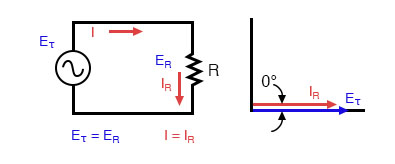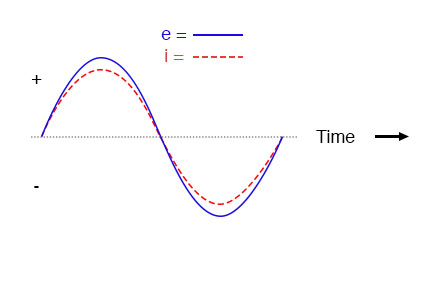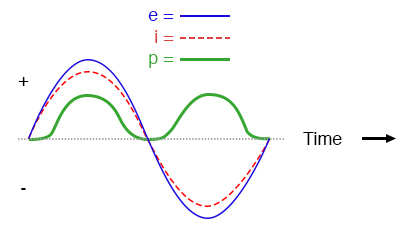# 3.1 AC Resistor Circuits (Inductive)Pure resistive AC circuit: resistor voltage and current are in phase.

If we were to plot the current and voltage for a very simple AC circuit consisting of a source and a resistor (figure above), it would look something like this: (figure below)Voltage and current “in phase” for resistive circuit.

Because the resistor simply and directly resists the flow of current at all periods of time, the waveform for the voltage drop across the resistor is exactly in phase with the waveform for the current through it. We can look at any point in time along the horizontal axis of the plot and compare those values of current and voltage with each other (any “snapshot” look at the values of a wave are referred to as instantaneous values, meaning the values at that instant in time). When the instantaneous value for the current is zero, the instantaneous voltage across the resistor is also zero. Likewise, at the moment in time where the current through the resistor is at its positive peak, the voltage across the resistor is also at its positive peak, and so on. At any given point in time along the waves, Ohm’s Law holds true for the instantaneous values of voltage and current.

We can also calculate the power dissipated by this resistor, and plot those values on the same graph: (figure below)Instantaneous AC power in a pure resistive circuit is always positive.

Back to Main Index of Book